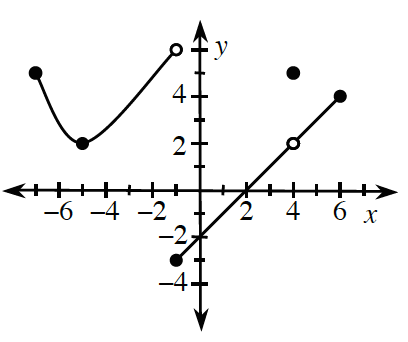### Home > CALC > Chapter 6 > Lesson 6.4.1 > Problem6-128

6-128.

The function $y = g(x)$ is graphed below.1. Find the domain and range.

The domain is closed. The range is not.

2. Where is the function continuous? (Hint: It is usually easiest to start with the domain and then eliminate where the function is not continuous.)

One point of discontinuity is $x = −1$.
Recall, that a function must meet three conditions to be considered continuous at a point:
1. The limit must exist. (That means the limit from the left must agree with the limit from the right.)
2. The actual value must exist.
3. The limit must agree with the actual value.

3. Where does the function appear to be differentiable?

To be differentiable:
1. Slope from the left must agree with slope from the right.
2. Differentiability implies continuity.
In other words, even if the slopes agree, a function cannot be differentiable where it is not continuous.